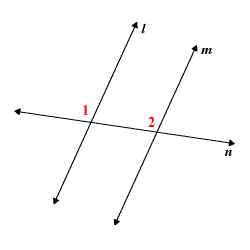# Corresponding Angles Postulate

The Corresponding Angles Postulate states that, when two parallel lines are cut by a transversal , the resulting corresponding angles are congruent .

So, in the figure below, if $l\parallel m$ , then $\angle 1\cong \angle 2$ .The converse is also true; that is, if two lines $l$ and $m$ are cut by a transversal in such a way that the corresponding angles formed are congruent , then $l\parallel m$ .